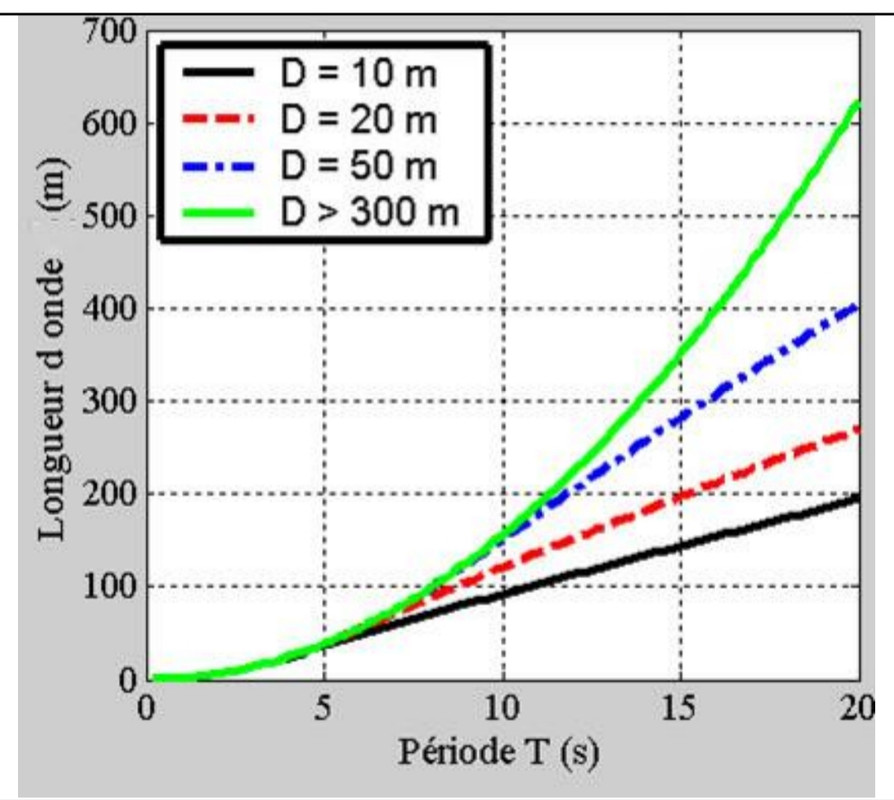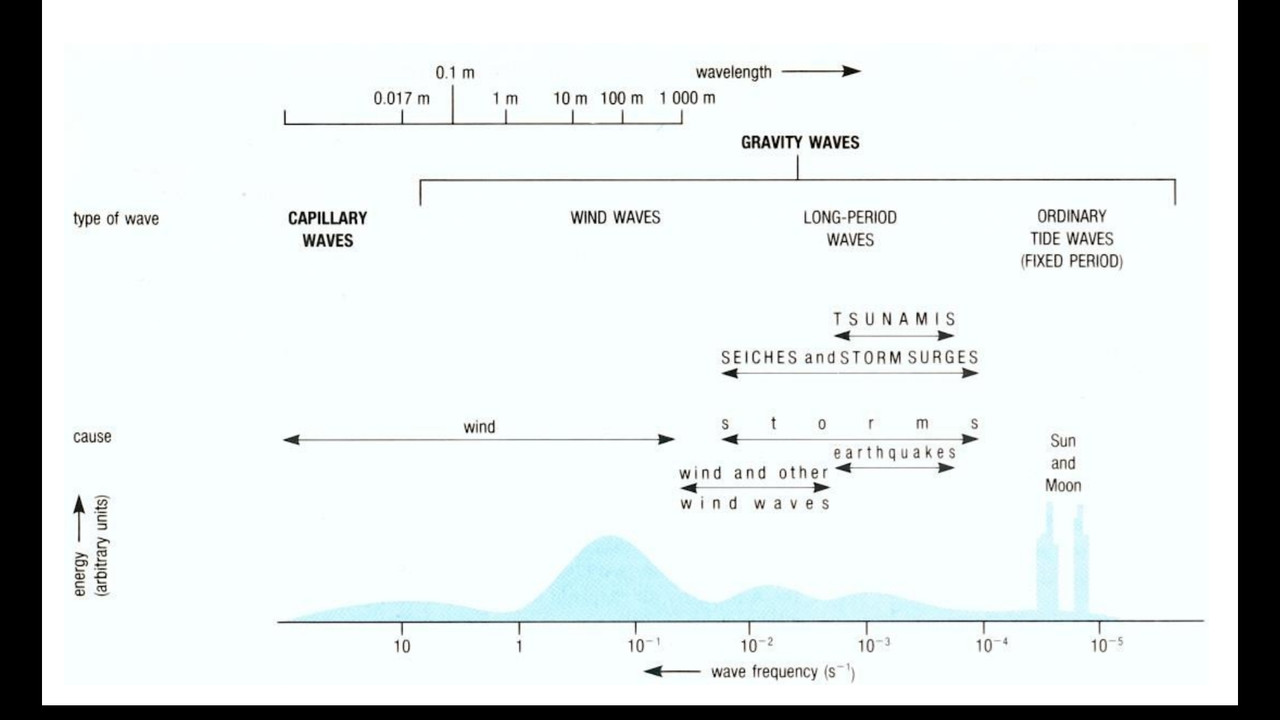# Wave length

• Is there a way to determine the length of waves (wavelength) based on wave height and frequency?

• @vsschreffler
You could obtain precisely the wavelength (distance between crest to crest) from the period (inverse of frequency) if you know the speed or celerity of waves. But the speed of waves is not constant (as it is for the sound in air). The waves speed depends on the wave length. The longer the waves are, faster is the swell.
Wave length in metres is about 1.6 times the square of their period in secondes (period is provided by Windy)
However it’s just a rule of thumb and only valid in deep water, not along the seashore !

As example an estimation of swell length on 100m depth water. (14 x 14 x 1.6 = 313m):• Well... That is an interesting question. Ocean waves are kind of complex. Of course, like any other waves, their speed, wavelength, period... Are connected. The other thing to take into account is the depth of the ocean or lake. There are other complex parameters such as the height of the wave. Two waves can have the same wavelength, but can vary in height... The higher one is more energetic and move slightly faster than the less energetic one, so their periods are different... So there is no linear rule between wavelength and period. This is not true for electromagnetic waves!

Moreover, equations are not simple and depend on the type of waves. For exemple, tsunami waves are not sinusoids... And I still can't understand why. However, this is a graph that can answer your question, valid for wind waves, showing different values depending on the depth of the ocean floor.L is wavelength in m
D is the depth in m
Period is in seconds

And this is an other graph showing frequencies of different ocean waves... You can see that tide waves are the most energetic ones! Their wavelengths can be over 6000km.